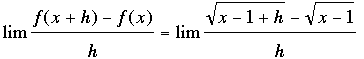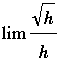SEARCH HOMEMath Central Quandaries & QueriesQuestion from edith, a student: describe the x-values at which f is differentiable. y= square root(x-1) explain your answer.Hi Edith,

Let's write f(x) = √(x - 1).

If x - 1 < 0 (that is x < 1) then √(x - 1) doesn't exist and hence x is not in the domain of the function. Hence f(x) = √(x - 1) is not differentiable if x < 1.

If x - 1 > 0 (that is x > 1) then x is in the domain of the function. To determine that f is differentiable at x you need to evaluateas h approaches zero.

Notice that since x - 1 > 0, if h > 0 then (x - 1 - h) > 0 and the square root exists. Also if h < 0 then for sufficiently small h, (x - 1 + h) > 0 and again the square root exists. Now you need to perform the algebra to determine if the limit exists.

The final possibility is x - 1 = 0. In this case the limit becomesas h approaches 0.

In this case when h < 0 the square root doesn't exist and hence the limit can't exist.

I hope this helps,
PennyMath Central is supported by the University of Regina and The Pacific Institute for the Mathematical Sciences.